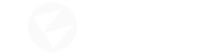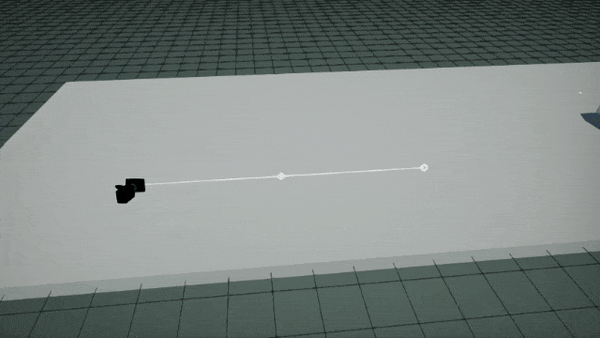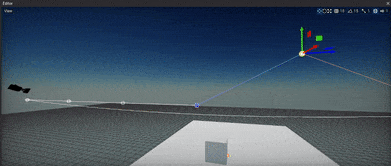# Animate actor linearly over spline

I’m trying make a camera path script that follow one actor target (player in most cases) using splines to draw the path. But `Spline.GetSplinePoint` give me a smooth movement by time.

This example reproduces that behaviour:``````currentTime += Speed * Time.DeltaTime;
var position = SplinePath.GetSplinePoint(currentTime);
CameraSlot.Actor.Position = position;
``````

Is it possible to interpolate the spline path linearly?

What are spline control points? If you set them to linear (empty tangents) then it should be linear spline, not the Bezier, right?

I think I understand now how splines works. When I set tangents to 0 this behavior of stopping at keyframe happens. Now I defined tangents positions to the same position of previous keyframe (for in tangent) and the next (for out tanget), then uniform movements happen.Tangents equal to 0 gets a smooth velocity moviment… tangents equal to the previous and next keyframes gets a hard and uniform movement that I want.

1 Like